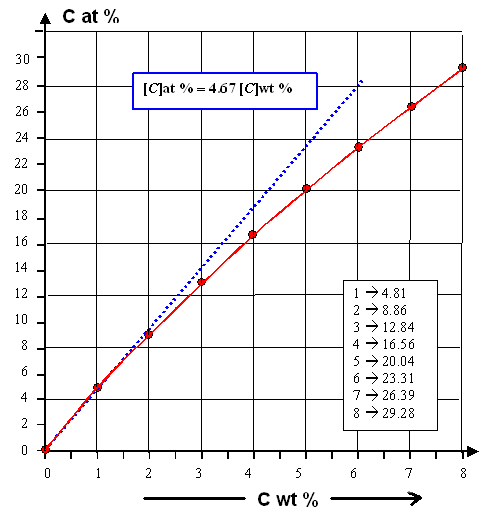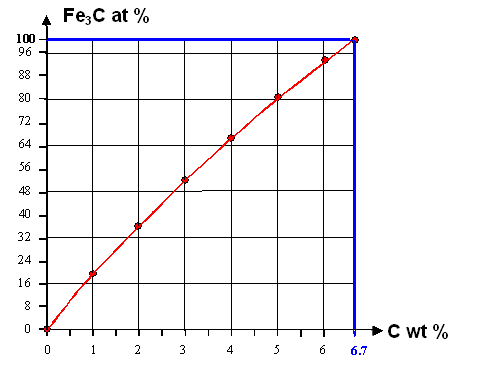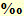# Numbers and Concentration of Atoms

General IssuesWhen we look at vacancies or carbon atoms inside an iron crystals, we can describe that qualitatively like: a lot of vacancies, a few vacancies, a substantial amount of carbon atoms, and so on. It's an easy description, giving you a general idea of what is at stake, but it is simply not good enough.
We need a quantitative way for looking at these things, and that means we need numbers.Giving the number of vacancies or carbon atoms in some piece of iron is an easy thing to do - provided you know the number. You may have, for example, about 2 000 000 000 000 000 000 000 000 carbon atoms in your steel blade, and that is obviously not a lot compared to the number of iron atoms in there, rouhly around 100 000 000 000 000 000 000 000 000.
Allright, you get the point: those numbers are a bit unwieldy—so we use the exponential notation. Now you have, for example, about 2 · 1024 carbon atoms inside the 1 · 1026 iron atoms of your steel blade.Is that a lot or rather not so many? How does this number compare to the number of carbon atoms in the steel wheel of a high-speed ICE train? That wheel weighs at least 100 times more then your sword blade. If it is made from the same steel, it must have at least 100 times more carbon atoms than your sword blade.You get my point once more: Precise numbers for the amount of carbon atoms or whatever in your iron are nice—but concentrations are nicer! Concentrations are always relative in the sense that you compare two numbers with one number as a reference.Concentrations are often given in percent (%) or as density. We only must be clear on one thing now: percent or density relative to what? Here are the options:
• You go for "weight percent" (wt %). That's the standard way, rather common and comfortable, since it is easy to weight things. 1 wt % carbon in iron means that 1 % of the total weight—iron plus carbon— is due to the carbon.
• You go for "atom percent" (at %). How many percent of all atoms (or molecules, particles, ...) of the material are carbon atoms?
• You go for volume percent. How much volume, in percent of the total volume, would be occupied by the carbon atoms? Not a very useful way to measure the carbon concentration in iron but not bad for giving the amount of oxygen in air, for example.
• You go for atoms / molecules / particles per volume unit, i.e. a density. The unit then is cm–3, i.e. number (no unit) per cubic centimeter.
• You go for atoms / molecules / particles per weight unit. The unit then is kg–3There is no "best" kind of concentration measure. What is best depends on your needs; what you want to do with the number.If you want to bake a cake, it might be best to give the concentration of sugar relative to the flour in weight percent. Actually, it is mostly done by volume, even omitting the percent: one cup of flour, two teaspoons of sugar. It certainly would be pointless to use molecule percent.But if you want to assess possible reactions between ingredients, it is far better to do atom percent. If you want to make water from scratch by reacting one atom of oxygen (O) with two atoms of hydrogen (H), i.e 2H + 1O = H2O, you should mix the oxygen atoms : hydrogen atoms = 1 : 2. Hydrogen atoms then account for 66,66 at % of the mix, the oxygen atoms supply the remaining 33,33 at %. Weight-wise that would be, for example, about 70 g of H to 533 g of oxygen, i.e. a relation of 1 : 7.6. The 70 g of H then would be 11.6 wt %.If you want to make iron carbide (Fe3C, also known as cementite) from scratch, you need one atom of carbon (C) and three iron (Fe) atoms, i.e 3Fe + 1C = Fe3C. You should mix carbon to iron 1 : 3, i.e. 25 at % C and 75 at % Fe.
Since a carbon atoms weighs 12 u = atomic units (or "Daltons"; with 1u = 1,66 ·10–27 kg), and an iron atom weighs about 56 u, weight-wise that would be about 24 · 12 = 300 g of C to 75 · 56 = 4.200 g of iron, i.e. a relation of 1 : 14.
The 300 g of carbon are thus about 6,6 wt % - but 25 at %

Carbon in IronThere is no simple relation between atom percent (at %) and weight percent (wt %), i.e. you can't just multiply one number with some constant to get the other. But there are simple equations. Here they are, together with graphs for the full range and for small carbon wt %.

[A]Bat %  =   A
A + B
· 100 %

[A]Bwt %  =  a · A
a · A + b · B
· 100 %

[A]B is the concentration of A in B.
A, B are the numbers of A and B atoms, resp., contained in the material.
a, b are the atomic weights of A and B atoms, respectivelyOf course, you could rewrite the first equation for B, and insert that in the second one to get some relation between [A]Bat % and [A]Bwt %. Do it! It's a good exercise in doing fractions. Here is the result:

[A]Bat %

=

100 %
[B]wt %  · a
[A]wt %  · b
+      1If we look at carbon (A = C) in iron (B = Fe), we have a / b = 12 : 56 = 0.21 and get

[C]at %

=

100 %
[Fe]wt %  · 0.21
[C]wt %
+    1Let's see how it works and assume we look at one weight percent (1 wt %) or two weight percent (2 wt %= carbon in iron. How much will that be in atom percent (at %)? Well, we have [C]wt % = 1 or 2, which leaves [Fe]wt % = 99 or 98. Multiplied by 0,21 it is 20.79 or 20.58. Dividing by 1 or 2 leaves 20.79 or 10.29. Adding 1 you get 21.79 or 11.29. Finally, 100 divided by 21.79 or 11.29 gives 4.81 at% and 8.86 at%For very small carbon concentrations, let's say below 1 wt %, we obviously get in a good approximation:

 [C]at % » 4.67 [C]wt %To save you a lot of calculations, I give you some graphs below that are more practical as far as carbon in iron is concerned.Here is the relation between carbon in weight percent (wt %) and carbon atom percent (at %). It is valid for all carbon atomically dissolved in the iron.Carbon in iron; weight and atom percent conversionHowever! We know that iron at room temperature can hardly dissolve any carbon. Instead an iron carbide is formed, It carries the name "cementite" and the chemical shorthand Fe3C. Upon carbide formation any carbon atom takes 3 iron atoms out of the "iron".
The curve below shows how much iron and carbon atoms are found in the cementite in at % for a carbon concentration given in wt %How much cementite in at % forms for a carbon concentration given in wt %Let's see how one should "read" this curve.
Let's assume that we have 2 wt % carbon in the iron. That corresponds to 8.86 at % (upper graph). Imagine that you have 10.000 atoms altogether; then we have 886 carbon atoms and 9.114 iron atoms. Now form cementite or Fe3C. That "eats up" 3 × 886 = 2.658 iron atoms that become part of the cementite, leaving only 9.114 - 2.658 = 6.456 iron atoms that still belong to the iron proper. The iron concentration is now only 65.56 at%, the cementite concentration accordingly 100 - 65.56 = 34.44 at%
The curve shows that for 2 wt%: The carbon and iron atoms in the cementite account for about 3 at %; leaving about 66 at% for the iron
Add 6.7 wt % carbon and it will eat up all the iron left. You would end up with pure iron carbide!

 DensitiesIt is very common and useful not to give concentrations in relative values or percent, but as volume density and (less frequently) as mass density. That means that instead of the concentration we now refer to the density rA; B of some particle A (atom, molecule, whatever) in some host B as Volume density: rA; B = A per cm3 or A cm–3. Mass density: r*A; B = A per kg or A kg–1. Those densities are often used if the numbers are very small or very large or if the "particles" are a bit more complex than simple atoms or molecules. Here are a few examples:Small concentrations: For example, the always very small impurity concentrations in silicon are mostly referred to like this riron  = 5 · 1013 cm–3 rP   = 2.38 · 1018 cm–3Large concentrations: The capacity of a battery, for example a lithium (Li) ion battery, is always given by how much Li you can "store" in the electrodes. A fully charged battery has all its Li in the anode; during discharge it moves to the cathode. When all the Li is in the cathode, the battery is empty and needs to be re-charged by moving the Li back into the anode. Numbers in percent (atom or weight) would be high and on occasion > 100 %. You can put more than 1 kg Li into a 1 kg Si anode, for example. There is a big difference between the volume density or mass density. If the battery is supposed to go into a car, weight matters far more than volume. If it is supposed to go into your basement to store you solar energy, it is the other way around.Complex particles: How do you give a number for the concentration / density of precipitates? You may care far more how many there are per volume than how big they are (needed for wt %). Giving atom % does not make much sense. Similar arguments go for other crystal lattice defects. If your "particle" is something more abstract, like the energy stored in a battery, you need to use densities. Energy has the unit "Watt hour" (Wh), and it is possible and easy to give the "specific capacity" in Watt hour per gram (Whg–1) or the energy density in Watt hour per cubic centimeter (Whcm–3). Obviously, percent scales are not useful here.
Small NumbersIf we don't discuss carbon in iron but for example vacancies in iron (or most other crystals), the maximum atomic concentration rarely exceeds 0.01 at % but can be far, far smaller.1 vacancy within 1.000.000 (1 mio) atoms would be a concentration of 0.0001 at %. Try to express that in weight percent!
Small numbers like that become cumbersome and error prone. So we do it by exponentials or by some frequently used abbreviations. I dealt with that already in this early module. Here is the important table from this module, what we need here is highlighted in pink. Use it but be careful about possible mix-ups with the billion / trillion confusion.

Large numbers   Small numbers
Potency Name Symbol Potency Name Symbol As "parts per .."
1024 Yotta Y 10–1 Dezi d
1021 Zetta Z 10–2 Zenti c %
percent
1018 Exa E 10–3 Milli mpromille
1015 Peta P 10–6 Mikro µ ppm
million
1012 Tera T 10–9 Nano n ppb
billion
109 Giga G 10–12 Piko p ppt
trillion
106 Mega M 10–15 Femto f ppqt
quatrillion
103 Kilo k 10–18 Atto a
102 Hekto h 10–21 Zepto z
101 Deka da 10–24 Yocto yGlossary5.2.2 Defects that Get Around5.2.1 The Gang of FourMyths and Bullshit Around Quenching9.1.1 Things are Complicated2.3.1 Let's get to Work.11.5 Wootz Swords; 11.5.1 The Winner is....Units of Length, Area, and VolumeExponentials and Logarithms6.1.1 It Takes Two to TangoProducing "Nirvana" Silicon or Nearly Perfect Silicon Single CrystalsIntrinsic Gettering in SiliconDisplacement and Strain

© H. Föll (Iron, Steel and Swords script)